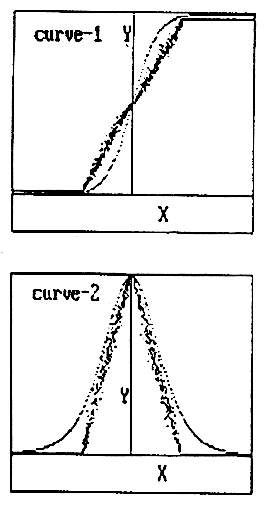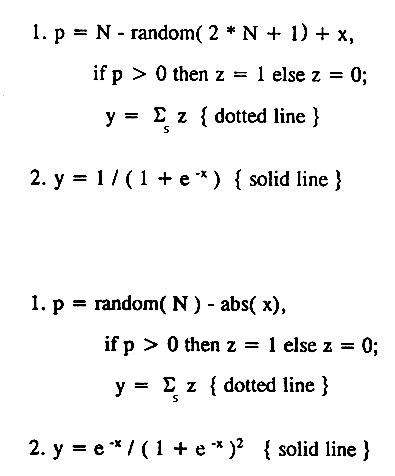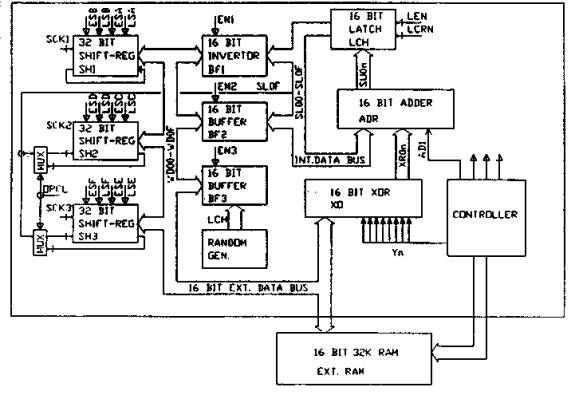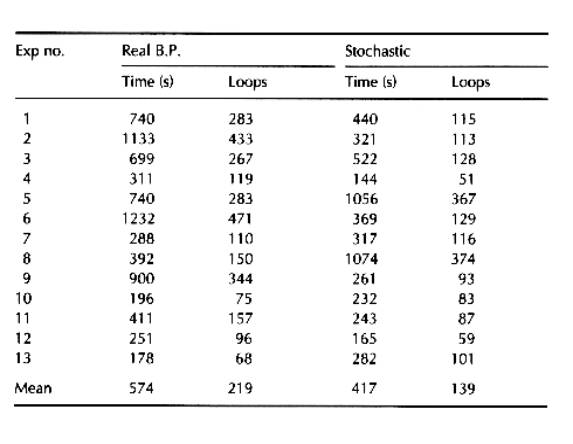## RENT A THINKER Home My Page Chat Tik-Tok 8        A MULTILAYERED FEED FORWARD NEURAL NETWORK SUITABLE FOR VLSI IMPLEMENTATION

### 8.1       Abstract

A potentially simplified training strategy for feed forward type neural networks is developed in view of VLSI implementation. The gradient descent back propagation technique is simplified to train stochastic type neural hardware. The proposed learning algorithm uses ADD, SUBTRACT, and LOGICAL operators only. This reduces circuit complexity with an increase in speed. The Forward and reverse characteristics of perceptrons are generated using random threshold logic. The supposed hardware consists of 31 perceptrons per layer working in parallel with a programmable number of layers working in sequential mode.

Keywords Stochastic, Multi layer, Neural Network, VLSI

### 8.2       Introduction

Multilayered Feed Forward Neural networks have created enormous interest among AI researchers for their feature extraction capability from input patterns. Their potential as problem solving tools has also been demonstrated with various learning algorithms. However, for any serial real world application, such networks should have a large number of interconnections operating in parallel, obeying predictable learning rules uniformly throughout the network. The VLSI implementation of such a network needs a large number of interface lines (pin connections). Learning algorithms like back propagation need large floating point operations, which increase rapidly with the increase in number of perceptrons per layer. It is thus necessary to give adequate stress to the simplification of the learning algorithm so as to reduce the computational need for massive parallelism.

This paper describes a simplified technique to implement multi layered Neural network using random number generators. The gradient descent technique is modified to implement stochastic logic. The prototype network built by us consists of maximum of 31 perceptrons in each layer. The learning algorithms uses only ADD and SUBTRACT and LOGICAL operations. Interlayer communication needs only one bit per output and hence minimizes the data path considerably.

### 8.3       Back Propagation Algorithm

We first review briefly the back propagation technique, which is normally used for training feed forward type neural networks. The input-output characteristic function of perceptrons is chosen such that it is continuous and its derivative is bounded between definite limits for a large dynamic range of input.

Consider a three layer network of units Yi, Yj and Yk interconnected through weights Wji and Wkj such that

Xj = Si Wji *  Yi;  Yi = f(Xj)                    and      Xk = Sj Wkj *  Yj;  Yj = f(Xk)

(First Layer)                                                   (Second Layer)

where Y = F(X) represents the input-output characteristic function of the perceptrons, which is usually chosen to be of the form:

f(x) = 1 / (1 + e –x ).

The weights Wji and Wkj are modified using the BP algorithm as follows.

Wkj = Wkj + n * Y * [(Yk – Dk) * Yk * (1 – Yk)}]                                                  (1)

Wkj = Wkj + n * Yj * {Yj * (1 – Yj)} * Skk [(Yk – Dk) * {Yk * (1 – Yk)} * Wkj]         (2)

Where n is a constant less than 1 and Dk is the desired output. Most of the operators in equations (1) and (2) operate on real variables. Hence it is difficult to implement a large number of perceptrons working in parallel in a VLSI.

It has been shown that the choice of the perceptron’s characteristics is not very critical for convergence of error during training. This paper suggests one such choice which reduces the computational requirement drastically without affecting the error convergence property during training.

### 8.4       Proposed Algorithm

We will analyze the significance of each factor in expressions (1) and (2) in order to replace them by suitable probability functions.

(Yk – Dk) is the departure of output Yk from the desired value Dk. Yk * (1 – Yk) is the measure of willingness of the Kth output perceptron to learn. This is measured by its nearness from its average value (0.5). This factor plays an important role in training. Wkj is the back propagation signal path’s conductance. Yj * (1 – Yj) is similar to the above term Yk * (1 – Yk) for a hidden layer perceptron. Yi is the excitation potential for the network.

In this architecture, we have considered all the perceptrons Yi, Yj and Yk having binary output. In the proposed system the perceptron’s forward pass transfer characteristics are generated by a threshold detector having a random bias value as shown in Figure 1. This is equivalent to a choice of input-output characteristic function Y = f(X) of the form

Y = 1 for (X + r) > 0

= 0 for (X + r) £ 0                                       (3)

where r is a random integer inside the dynamic range of X. Yj and Yk are calculated using the above expression in the forward pass. This has two advantages: the value of Yj is binary but is statistically continuous in the dynamic range of learning and the computation of Xk (for the next layer) does not need any multiplications.

In the backward pass, a term of the form Yk * (1 – Yk) is desirable. This term increases the sensitivity of Wkj near the threshold value of Xk. Equivalently; the network’s willingness to learn or forget is inversely proportional to the distance of Xj from the threshold. Considering this, a simplified reverse characteristic function R(X) as shown in figure is used to replace the terms of the form Yk * (1 – Yk) in equations (1) and (2).

R(X) = 0 for abs (X) > r

= 1 for abs (X) £ r                                                         (4)

where r is a random integer in the range 0 £ r < N and the dynamic range of X for learning is (-N to +N).Figure 1: The forward and reverse characteristics of the proposed stochastic function along with the exponential sigmoid function (solid line).

The value of N is dependent on the network configuration. To improve the dynamic range of inputs and the rate of convergence the following options are listed.

·        Use of N as random integer itself;

·        Use of r as a weighted random number;

### 8.5       Hardware Description

Figure 2 shows a simplified hardware diagram of the multi-layered neuroengine. The system consists of three 32 bit registers, one 16 bit adder and a random number generator which are connected to memory via a 16 bit bus. A PROM based sequence controller generates all necessary control signals for different passes and is also used to store the configuration of the network.

Register SH1 is loaded with input Yi, which is rotated 32 times using 1024 clock cycles. In every clock cycle let the corresponding weight Wji is fetched from external RAM and depending on the MSB of SH1, Wji is added to or subtracted from the contents of the latch. After one complete cycle of SH1 Xj is computed in LCH. In training mode a random number is added to Xj through BF3. The sign bit of Xj represents Yj which is stored in SH3. All Yj are computed from Wji and Yj in 1024 clock cycles.

The learning mode needs five passes for a two-layer network as follows.

1.      Compute Yi

2.      Compute Yk

3.      Correct Wkj

4.      Compute Dj

5.      Correct Wji

The complete two layer learn cycle needs about 7000 clock cycles, which less than one millisecond.

### 8.6       Results

The above algorithm is first tested in a simulated environment using 16bit integer arithmetic. The results are compared with those from the original back propagation algorithm using real number arithmetic. An IBM-386 computer with co-processor was used for testing both the methods. Both the networks were configured with eight input units, eight hidden units, and one output unit. All parameters in both types of networks were optimized for minimum convergence time. The output function was chosen as mirror symmetry detection of input data. The convergence success rate was found to be larger and convergence time shorter in the proposed algorithm as compared to the BP algorithm. Some sample results are shown in table 1. TTL based hardware has also been tested for successful convergence for various output functions.Figure 2: Block diagram of the proposed multi layered feed forward neural network.

Table 1: Sample Results.### 8.7       Appendix

A sample program for three-layer network, N0, N1, N² and N³ are constants adjusted for dynamic range and rate of convergence.

PROCEDURE forward_pass;
BEGIN

FOR j:=0 TO jmax DO

BEGIN

xj[j]:=0;

FOR i:=0 TO imax+1 DO

IF yi[i]>0 THEN xj[j]:= xj[j] + wji[j,i];

IF xj[j]>random(N0)  THEN yj[j]:=1  ELSE yj[j]:=0;

END;

FOR k:=0 TO kmax DO
BEGIN
xk[k]:=0;
FOR j:=0 TO jmax+1 DO
IF yj[j]>0 THEN xk[k] := xk[k] + wkj[k,j];
IF xk[k]>random(N0)  THEN  yk[k]:=1  ELSE  yk[k]:=0;
END;
END;
PROCEDURE training_pass;
BEGIN
FOR j:=0 TO jmax+1 DO outj[j]:=0;
FOR k:=0 TO kmax DO
BEGIN

IF abs(xk[k]) > random(N1) THEN p:=0 ELSE p:=1;
d:=out[k]-yk[k];

IF d<>0 THEN
FOR j:=0 TO jmax+1 DO
BEGIN
IF yj[j]>0 THEN wkj[k,j] := wkj[k,j] +d
ELSE wkj[k,j] := wkj[k,j] -d;

IF p>0 THEN
IF d>0 THEN outj[j]:=outj[j] + wkj[k,j]

ELSE outj[j]:=outj[j] - wkj[k,j];

END;

END;
FOR j:=0 TO jmax DO
BEGIN

IF abs(xj[j]) > random(N2) THEN a:=0 ELSE a:=1;

IF abs(outj[j]) > random(N3) THEN b:=1 ELSE b:=0;

d:=a and b;

IF d<>0 THEN

IF outj[j]>0 THEN

FOR i:=0 TO imax+1 DO

IF yi[i]>0 THEN wji[j,i] := wji[j,i] +d

ELSE wji[j,i] := wji[j,i] -d

ELSE

FOR i:=0 TO imax+1 DO

IF yi[i]>0 THEN wji[j,i] := wji[j,i] -d

ELSE wji[j,i] := wji[j,i] +d;
END;
END;

### 8.8       References

 Rumelhart D.E., Hinton G.E., and Williams R.J., Learning Representations by Back-Propagation Errors. Nature, Vol.323, No. 9, pp.533-536, Oct.1986.

 David S. Touretzky and Dean A. Pomerleau, "What's Hidden in the Hidden Layers?", BYTE, pp.227-233, August 1989.

 Widrow B., Winter R.G., and Baxter R.A., (1988). Layered Neural Nets for Pattern Recognition, Transactions on Acoustics, Speech and Signal Processing, vol. 36 No. 7 July 1988.

  Lang,K.J.,  Waibel,A.H., and Hinton G.E.,  A Time-Delay Neural Network Architecture for Isolated Word Recognition. Neural Networks, Vol.3, pp.23-43, 1990.

 Alspector, J.Allen, R.B.,Hu.V., & Satyanarayana, S., Stochastic learning networks and their implementation. In D.Z. Anderson (Ed.), Proceedings of the IEEE Conference on Neural Information Processing Systems - Natural and Synthetic, pp.9-21, New York American Institute of Physics, 1988.

 Gagan MirchandanI and WeI Cao, "On Hidden Nodes for Neural Net", IEEE Transactions on Circuits and Systems Vol.36, No.µ May 1989.

 Lippmann R.P., An introduction to computing with Neural Nets. IEEE ASSÐ Magazine, pð 4-22, April,1987.

 W P. Jones and Josiah Hoskins, "Back-Propagation” BYTE, pp.155-162, October 1987.

8.9 Publication

Himanshu S. Mazumdar, " A VLSI implementation of multilayered feed forward neural network ", published in the international journal  -Microprocessors and Microsystems, vol.- 19, number-4, May, pp.231-235, USA, 1995.